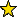# Quasidegrees -- a package to compute quasidegrees

## Description

Quasidegrees is a package that enables the user to construct multigraded rings and look at the graded structure of multigraded finitely generated modules over a polynomial ring. The quasidegree set of a $\ZZ^d$-graded module $M$ is the Zariski closure in $\CC^d$ of the degrees of the nonzero homogeneous components of $M$. This package can compute the quasidegree set of a finitely generated module over a $\ZZ^d$-graded polynomial ring. This package also computes the quasidegree sets of local cohomology modules supported at the maximal irrelevant ideal of modules over a $\ZZ^d$-graded polynomial ring.

The motivation for this package comes from $A$-hypergeometric functions and the relation between the rank jumps of $A$-hypergeometric systems and the quasidegree sets of non-top local cohomology modules supported at the maximal irrelevant ideal of the associated toric ideal as described in the paper:

Laura Felicia Matusevich, Ezra Miller, and Uli Walther. Homological methods for hypergeometric families. J. Am. Math. Soc., 18(4):919-941, 2005.

This package requires FourTiTwo, Depth, and Polyhedra.

## Caveat

This package is written when the ambient ring of the modules in question are positively graded and are presented by a monomial matrix, that is, a matrix whose entries are monomials. This is due to the algorithms depending on finding standard pairs of monomial ideals generated by rows of a presentation matrix.

## CertificationVersion 1.0 of this package was accepted for publication in volume 9 of The Journal of Software for Algebra and Geometry on 26 February 2019, in the article Computing quasidegrees of A-graded modules. That version can be obtained from the journal or from the Macaulay2 source code repository.

## Version

This documentation describes version 1.0 of Quasidegrees.

## Source code

The source code from which this documentation is derived is in the file Quasidegrees.m2.

## Exports

• Functions and commands
• Methods
• "exceptionalSet(Matrix)" -- see exceptionalSet -- returns the exceptional set of a matrix
• "quasidegrees(Ideal)" -- see quasidegrees -- compute the quasidegree set of a module
• "quasidegrees(Module)" -- see quasidegrees -- compute the quasidegree set of a module
• "quasidegreesAsVariables(Ideal)" -- see quasidegreesAsVariables -- represents the quasidegree set in variables
• "quasidegreesAsVariables(Module)" -- see quasidegreesAsVariables -- represents the quasidegree set in variables
• "quasidegreesLocalCohomology(Ideal)" -- see quasidegreesLocalCohomology -- returns the quasidegree sets of local cohomology modules
• "quasidegreesLocalCohomology(Module)" -- see quasidegreesLocalCohomology -- returns the quasidegree sets of local cohomology modules
• "quasidegreesLocalCohomology(ZZ,Ideal)" -- see quasidegreesLocalCohomology -- returns the quasidegree sets of local cohomology modules
• "quasidegreesLocalCohomology(ZZ,Module)" -- see quasidegreesLocalCohomology -- returns the quasidegree sets of local cohomology modules
• "removeRedundancy(List)" -- see removeRedundancy -- removes redundancies from a list of planes